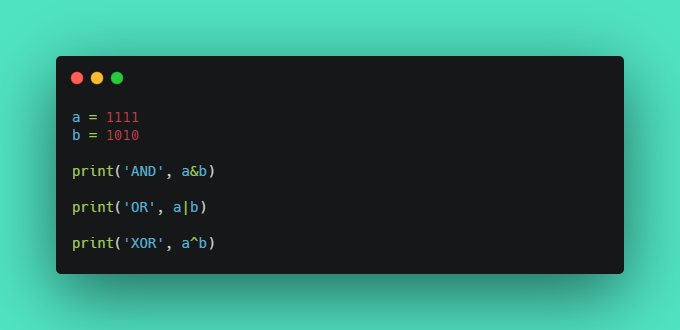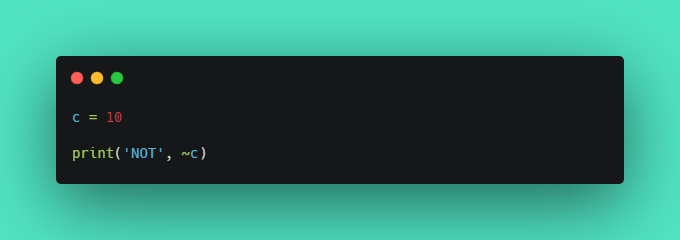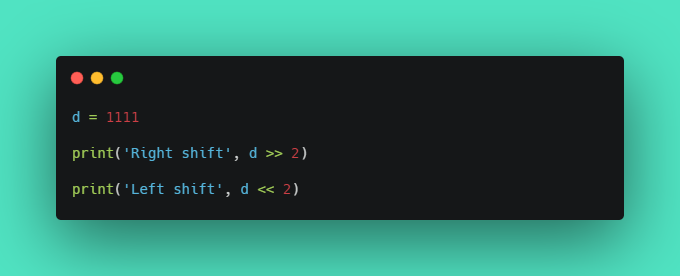Bitwise Operators in python

When it comes to binary numbers, bitwise operators are the choice.

Bitwise operators are used to performing operations on binary numbers.

AND, OR, XOR operators

• AND & operator sets each bit to 1 if both bits are 1.
• OR | operator sets each bit to 1 if one of two bits is 1.
• XOR ^ operator sets each bit to 1 if only one of two bits is 1.Output:
AND 82
OR 2039
XOR 1957

Ha Ha, surprised about the outputs?! The outputs are a result of the binary numbers a and b which gets converted into an integer, each time bitwise operation is performed.

NOT operator

• NOT ~ operator inverts all the bits.
• In python, the number gets converted into an inverted signed number.Output:
NOT -11

Shift operators

• left shift << operator shifts left by pushing zeros in from the right and let the leftmost bits fall off.
• right shift >> operator shifts right by pushing copies of the leftmost bit in from the left, and let the rightmost bits fall off.Output:
Right shift 277
Left shift 4444

Code along and have fun ;)Aswin Barath

My name is Aswin Barath. I’m a technical blogger and budding software engineer